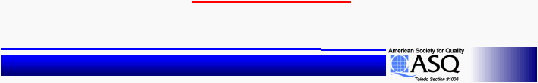< > unit5ReadOnly - page 7 of 13 Six Sigma Six Sigma Training - 6 SigmaStatistical Design of Experiments
Statistical Design of Experiments
Reference
Reference
F value:
F value:
The variance ratio is used to determine whether the
The variance ratio is used to determine whether the
variance of a factor is significantly greater than that
variance of a factor is significantly greater than that
represented by the error variance.
represented by the error variance.
S’ (Pure Sum of Squares):
S’ (Pure Sum of Squares):
This column is a refinement to the Sum
This column is a refinement to the Sum
of Squares.  It is an adjusted standard deviation without
of Squares.  It is an adjusted standard deviation without
reflecting error contribution.
reflecting error contribution.
S’ = Sum of
S’ = Sum of
Squares
Squares
Factor
Factor
-
-
Pooled Error*DF
Pooled Error*DF
Factor
Factor
Rho%:
Rho%:
%:
The percent contribution of the source to the total variance
The percent contribution of the source to the total variance
of the process.
of the process.
Rho% = Pure Sum of Squares * 100
Rho% = Pure Sum of Squares * 100
Total Sum of Squares
Total Sum of Squares